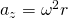# When was a direction established

## Forces in circular movements

### Measuring the centripetal force

The following experimental set-up is used to measure the centripetal force on a rotating body:A rail on which a carriage is located is set in rotation with the help of a motor. The carriage is connected to a force gauge via a pulley so that the necessary centripetal force can be measured.

By changing the height of the force sensor, the radius (= Distance between the center of gravity of the car and the center of the circle, see illustration). The radius can be read off with the help of the pointer and the scale on the rail.

To the Dimensions To change the trolley, it can be equipped with additional weights.

The Orbital period is determined with the help of a light barrier: The time required for each cycle is displayed directly on the light barrier - a mean value can thus be formed from several measured values.

#### Series of measurements

In order to determine the dependence of the centripetal force on the above-mentioned quantities, in each case a of these sizes varies, the others must be kept constant.

It will three series of measurements carried out:

1. Determination of the centripetal force as a function of Radius r

The centripetal force is at constant rotational frequency f or period of rotation T and constant mass m determined for different radii r:

m = 150g = 0.15kg

T = 0.8s f = 1.25Hz

 r in cm 10 13 16 19 25 F.z in N 0,9 1,25 1,5 1,8 2,3

2. Determination of the centripetal force as a function of the Rotation frequency f

The centripetal force is at constant mass m and constant radius r determined for different rotational frequencies f:

m = 150g = 0.15kg

r = 25cm = 0.25m

 f in Hz 0,63 1,04 1,25 1,56 1,85 F.z in N 0,6 1,6 2,3 3,6 5,1

3. Determination of the centripetal force as a function of the Mass m

The centripetal force is at constant rotational frequency f and constant radius r intended for different masses m:

r = 20cm = 0.2m

T = 1s f = 1Hz

 m in kg 0,1 0,15 0,2 0,25 0,3 F.z in N 0,8 1,2 1,6 2,0 2,4

### Test results and evaluation

The following relationships result from the measurements:

1. The centripetal force is proportional to the radius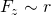2. The centripetal force is proportional to square the rotational frequency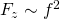Since the Angular velocity ω is proportional to the frequency f (the following applies:), the centripetal force must also be proportional to the square of the angular velocity.

The centripetal force is proportional to the square of the angular velocity: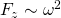3. The centripetal force is proportional to the mass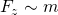#### Summary of the proportionalities

From the relationships 1., 2. and 3. it follows: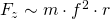or.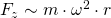The quotient from both sides is therefore constant: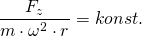or.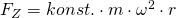If you put the units of all sizes, you can see:

All units can be shortened. So the constant has no unit.

If you determine the Numerical value of the constants from the series of measurements, the result is approximately the value 1. So you can simply leave them out and you get the formula you are looking for:

Formula for calculating the centripetal force: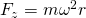To determine the centripetal force as a function of the Track speed to be able to calculate

we use the context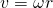or.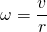and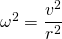Inserted into the formula for the centripetal force (see above), this results in another formula:

Formula for calculating the centripetal force: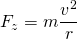For the Centripetal acceleration az applies:or.